# Animating Multidimensional Scaling to Visualize Large N-Dimensional Data Sets

Chris L. Bentley
Computer Science Department
Worcester Polytechnic Institute

Overview

Goal: Present enhancements to Multidimensional Scaling (MDS) to extend technique to large data sets (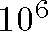points) with many dimensions (up to 50)

• What is N-dimensional data?
• How can we analyse it?
• How can we visualize it?
• Focus on one technique: Multidimensional Scaling
• Enhancements to traditional MDS: MAVIS
• Conclusions & Future Work

Visualization

• Science produces a numeric representation of our world
• We search for: patterns, clusters, anomalies, outliers, things which make a difference to us.
• Raw numeric data is difficult to interpret
• Scientific Visualization translates numbers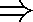Images
• This plays to the innate strengths of human visual perception

What is N-dimensional data?

We are familiar with 2 dimensional data defined by coordinate pairs (x,y). The Cartesian plane makes axes explicit, depicting entire data space. We can infer coordinate values of each point from the axes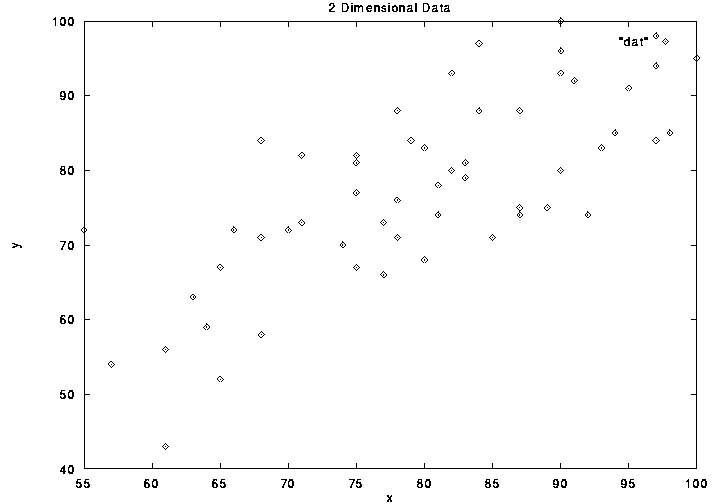2-column table makes values explicit - leaves us to imagine data space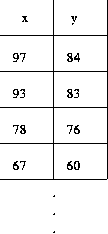N-dimensional data consists of points in a space with N coordinate axes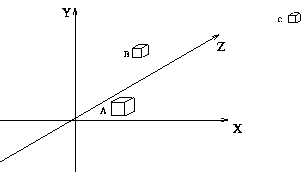In table form, N-dimensional data is a mxn matrix. Each row i defines a data point and is an n-vector. Each column is an axis or dimension.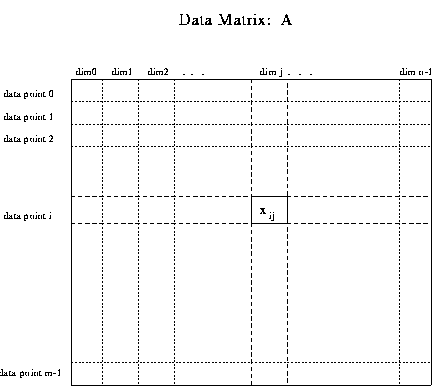Analysing N-Dimensional Data

How do we find information in an mxn matrix of numbers?

• Statistical methods - reduce data to summarizing values: mean, variance of columns or of entire matrix, measures of correlation or covariance between dimensions.
• Multivariate regression analysis - finds the line, plane, hyperplane which summarizes the data with least error
• Cluster analysis - groups data points with closest n-vectors, reduces number of data points. Cluster centroids can be treated as proxy data points

Visualizing N-Dimensional Data

How do we picture data with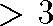dimensions? There are four basic approaches

• look at only a subset of the dimensions (e.g. all pairs) Multiple views can be displayed simultaneously - scatterplot matrices
• rearrange axes so that they are no longer orthogonal - star glyphs, parallel coordinates, dimensional stacking (XmdvTool)
• provide interactive manipulation of the data, so that user can explore data from multiple perspectives (PRIM-9)
• reduce dimensionality of the data so 2D/3D graphics techniques can be used

Reducing Dimensionality

Goal: to reduce mxn matrix to mxp, where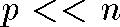Several methods can be used:

• Factor Analysis - finds subset of columns (dimensions) which explain majority of variance in all columns of matrix
• Principal Component Analysis - finds subset of columns (dimensions) which explain majority of correlation in all columns of matrix
• Dimensional Clustering - combine dimensions with greatest correlation - each iteration reduces dimensionality by 1
• Kohonen Self Organizing Feature Maps - uses neural network to arrive at matrix of weights that summarizes all n columns
• Multidimensional Scaling (MDS) - developed between 1938 - 1962 by Richardson, Torgerson, Shepard, Kruskal. Initially used for displaying multivariate data from psychological testing

Multidimensional Scaling: Central Idea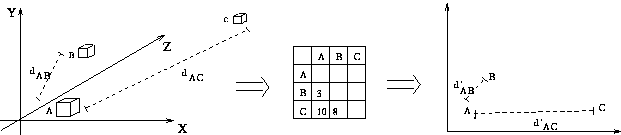• measure all interpoint distances in n-dimensional data space
• place 2D display points so they obey same mutual distance relationships

Is a perfect 2D configuration always possible? No.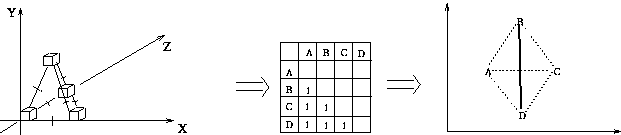Distances

• distances in any dimension are always scalar values - they are one dimensional, thus they provide a bridge from nD to 2D
• similarity data is often analogous to distance
• 2D distance formula is familiar Pythogorean Theorem: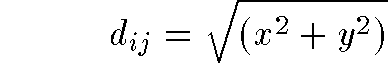• generalized distance formula in n-dimensions: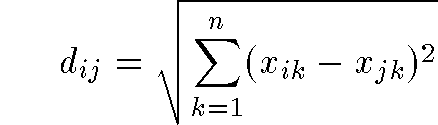• even more general Minkowski metric: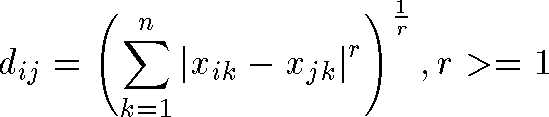Stress

• If the nD distance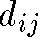equals the 2D distance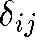then points i and j are correctly placed relative to one another
• If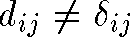then there is stress between the data and the 2D representation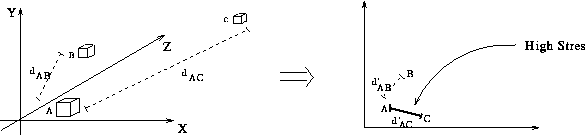• For all points in data set: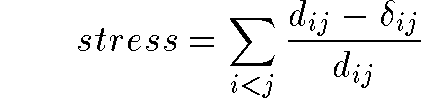• Or the variant: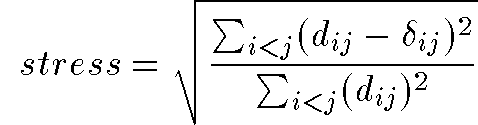Minimizing Stress

• MDS seeks a configuration of 2D display points that is a global minimum of stress
• This can be calculated using: gradient descent, simplex method, simulated annealing, or other optimization techniques
• Equivalent technique is the force based vector approach:
```1       calculate nD distances between data points
2       generate starting set of 2D display points
3       calculate 2D distances between display points
4       while stress decreases
4.1         calculate stress for each distance pair
4.2         use stress to scale vector between points
4.3         update point positions by summing vectors```

Figure shows vectors acting on point A, and cummulative vector in which point A will be updated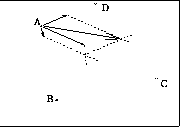Measuring MDS

• After t iterations of MDS, points will have moved into a low stress configuration
• How good is a given MDS solution? How do we measure this?
1. Shepard plot - 2D distances vs. nD distances
2. Stress plot - graph of overall stress should drop
3. Correlation plot - graph of correlation between 2D distances and nD distances should approach 1.0 Calculated using Pearson's r: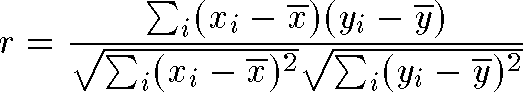• Figure 1. shows Shepard plot
• Figure 2. shows Stress graph
• Figure 3. shows Correlation graph
Problems With MDS

• Between m data points there are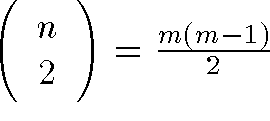distance relationships
• Thus stress is a function of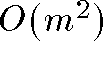variables
• Time complexity: each iteration of MDS is quadratic. In total MDS is between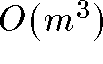and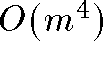• Space complexity: to store the distance matrices fordata points would require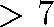terabytes of memory (7 million megabytes)
• As data dimensionality increases MDS has more trouble finding a global minimum of stress.

Solution #1: Subset-MDS

How to extend MDS so that it can be used with large data sets?

• If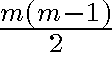distances are the problem don't use them all!
• Use only a subset of all distances: Subset-MDS
• If each point maintains distance relations to k others MDS will use only km distances - linear space, time complexity
• Subset-MDS sacrifices some accuracy for tractability

Questions:

• How should we select which k distances to retain?
• For given k, how well does Subset-MDS approximate MDS?

Subset-MDS: Selecting Distances

Subset-MDS could select k random distances for each pointOr, Subset-MDS could retain closest k/2 & farthest k/2 distances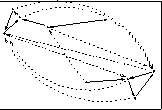• First Figure shows starting configuration of 5-dimensional data on iris flowers mapped to 3D. Dimensions with largest variance are used to initialize display coordinates
• Second Figure shows Subset-MDS solution using closest k/2 & farthest k/2 distances
• Third Figure shows Subset-MDS solution using random distance selection
Subset-MDS vs. MDS

• The Figure below shows that the correlations achieved by Subset-MDS improves as the subset size grows.
• In fact k = 10 achieves good correlations
• This is probabaly due to ``transitive relationships'' between data points
• If A is near to B and B is near to C then A will be placed near C for free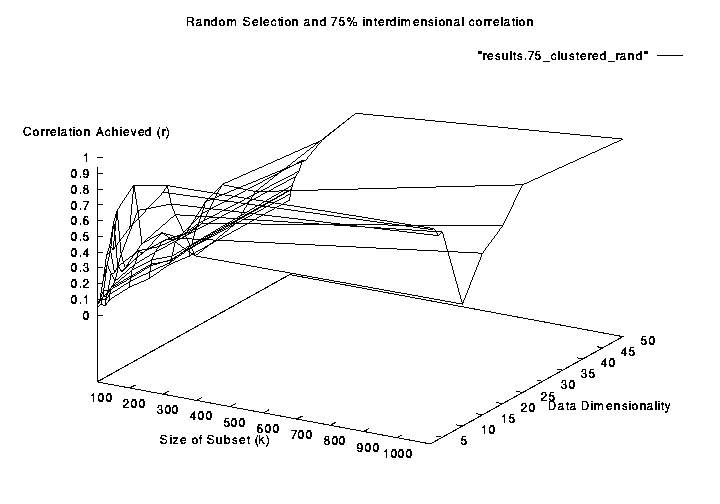Solution #2: Animation + Randomness

• As data dimensionality increases MDS cannot achieve low stress solutions
• Rather than let MDS terminate in a poor local stress minimum, why not add random noise to point positions to keep stress high?
• This will prevent MDS from equilibrating, and it will escape local minima, or at least cycle between a set of neighboring minima
• We will get more information from this cycling than from static
• This method similar to the simulated annealing method for escaping local minima equilibrium

• Figure shows points of 3D tetrahedron animating towards low stress configuration in 2D display space.
• Red indicates points are highly stressed
Random Perturbation

Random noise can be added to 2D display point coordinates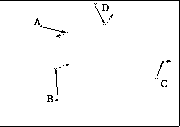Or point updates can be scaled so points overshoot low stress position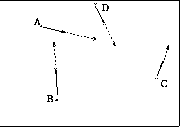It may be necessary to interpolate between successive positions

• Figure shows points of 3D cube trapped in a high stress configuration.
• Rerunning same MDS procedure with random perturbation of display points achieved lower stress solution
Conclusion

Summary

• MDS was briefly introduced
• Subset-MDS was proposed as linear time O(m) approximation
• Initial results indicate approximation to MDS is good
• Animating MDS with random perturbation was proposed
• More research needs to be done on this

Future Work

• Implement gradient descent MDS for comparison
• Implement simulated annealing MDS for comparison
• Research on adaptive variants of Subset-MDS
• Adding clustering to preprocess large data sets